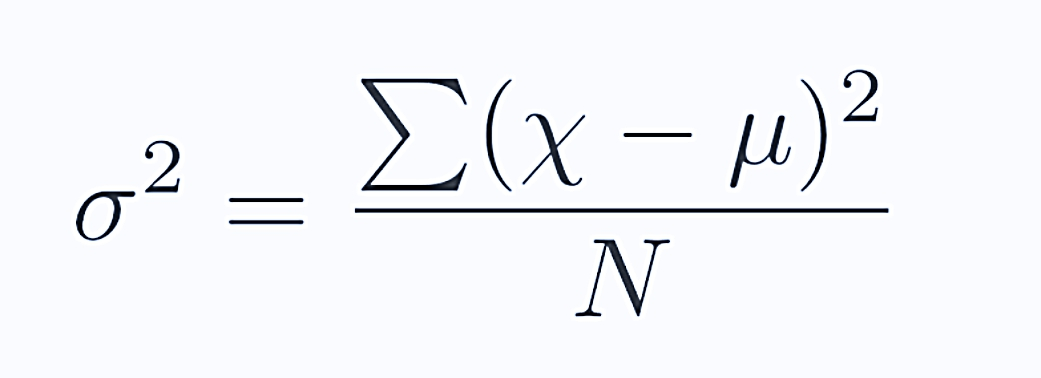--># These are the only importance of variance in statistics

## These are the only importance of variance in statistics

The point of statistics is to represent some situation (quantified, ostensibly, in a data set) in an efficient, concise, and clear manner. What the “typical” member of the set appears like, and how similar the objects are. Statistics in a sense had been created to represent the data in two or three numbers.

The variance is a measure of how dispersed or spread out the set is, some thing that the “average” (mean or median) is now not designed to do. If I inform you a data set has a variance of zero, for example, you know that each member of it is the same. If the variance is high, specifically as a ratio to the mean, that tells you that the data have some very massive dis-similarities amongst its members.

When assessing a set of numbers, in statistical inference, we talk about “moments.” those calculations that derive from the data themselves.

The first moment is the “mean.” It is, in layman’s terms, a description of the location of the data: the centre of where the points are.

The second moment is the “variance.” It is a description of how spread the data are - if one considers the mean to be a sort of centre of the data, the variance tells you how scattered around the centre of mass the points are.

The third moment is the “skewness.” It is itself a description of how balanced the data are. Do they cluster in mass to the left with a few points way off to the right, or are they symmetrical. A data set that is equally distributed to the left and right has zero skewness. The data for income in the US are heavily skewed.

There are others, but these three are in most realistic cases to the common man, enough to tell you most of what you need to know about what the data look like:

1. Where are they

2. How spread out (similar or dis-similar) are they

3. How balanced are they?